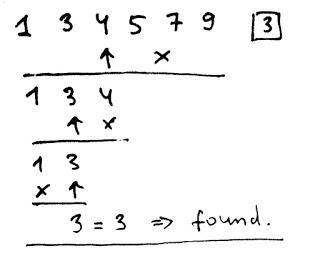# 通过 Lisp 语言理解编程算法：数组篇（上）CL-USER> (make-array 3)#(0 0 0)

CL-USER> (make-array 3 :element-type 'list :initial-element nil)#(NIL NIL NIL)CL-USER> (make-array 3 :initial-contents '(1.0 2.0 3.0))#(1.0 2.0 3.0)

CL-USER> #(1.0 2.0 3.0)#(1.0 2.0 3.0)

CL-USER> (let ((vec (make-array 3 :initial-contents '(1.0 2.0 3.0))))           (print (aref vec 0))           (print (? vec 1))           (:= (aref vec 2) 4.0))           (print (? vec 2))           (aref vec 3))1.0 2.0 4.0; Evaluation aborted on #<SIMPLE-TYPE-ERROR expected-type: (MOD 3) datum: 3>

CL-USER> (let ((vec #(1.0 2.0 3.0)))           (:= (aref vec 2) nil)           (print vec)); caught WARNING:;   Destructive function (SETF AREF) called on constant data.;   See also:;     The ANSI Standard, Special Operator QUOTE;     The ANSI Standard, Section 3.2.2.3; ; compilation unit finished;   caught 1 WARNING condition #(1.0 2.0 NIL)

RUTILS 提供了更多的选项，可以用缩写符号轻松创建数组：

CL-USER> #v(1 2 3)#(1 2 3)CL-USER> (vec 1 2 3)#(1 2 3)

## 数组作为序列

• 查询序列的长度：使用函数 length 在 Lisp 中执行。
• 通过索引访问元素：RUTILS ? 操作符是最通用的变体，而数组的原生变体是 aref，对于所有内置序列，则是更为通用的 elt（这还包括列表，在某些实现中，还包括用户定义的所谓的可扩展序列）。
• 获取子序列：该标准为此目的提供了函数 subseq

• 对于数组，length 函数在 O(1) 时间内工作，因为长度在数组结构中被跟踪。有一种替代（更原始）的方法来处理数组，主要是在 C 语言中，当长度没有存储时使用这种方法。相反，还有一个特殊的终止符用于表示数组的结束。例如，在 C 语言中，字符串有一个 \0 终止符，而在 Unix 系统调用 API 中，表示命令行参数的数组（如函数 exec）则是以空指针来终止的。首先，从算法的角度来看，这种方法效率并不高，因为查询数组长度需要 O(N) 个时间。但是，更重要的是，它已经被证明是许多灾难性安全漏洞的来源：古老的“缓冲区溢出”系列错误。

• subseq 函数创建其参数部分的副本，这是一个开销很大的操作。这是一种适当的默认函数式方法，但许多算法并不涉及子数组变化，对它们来说，更有效的变体是使用共享结构变体，这个变体不进行复制，而只是返回指向原始数组的指针。在 Lisp 标准中，这种选项是通过所谓的替换数组来提供的，但使用起来有些麻烦，这就是为什么在 RUTILS 中提供了更简单的版本，名为 slice

CL-USER> (with ((vec (vec 1 2 3))                (part (slice vec 2)))           (print part)           (:= (? part 0) 4)           (print part)           vec) #(3)#(4)#(1 2 4)

## 动态向量

(defun map-vec (fn vec)  "Map function FN over each element of VEC   and return the new vector with the results."  (let ((rez (make-array (length vec))))    (dotimes (i (length vec))      (:= (aref rez i) (call fn (aref vec i))))    rez)) CL-USER> (map-vec '1+ #(1 2 3))#(2 3 4)

(defun clumsy-filter-vec (pred vec)  "Return the vector with only those elements of VEC   for which calling pred returns true."  (let ((rez (make-array (length vec) :fill-pointer t)))    (dotimes (i (length vec))      (when (call pred (aref vec i))        (vector-push (aref vec i) rez)))    rez)) CL-USER> (describe (clumsy-filter-vec 'oddp #(1 2 3)))#(1 3)  [vector]Element-type: TFill-pointer: 2Size: 3Adjustable: yesDisplaced-to: NILDisplaced-offset: 0Storage vector: #<(SIMPLE-VECTOR 3) {100E9AF30F}>

CL-USER> (let ((vec (make-array 0 :fill-pointer t :adjustable t)))           (dotimes (i 10)             (vector-push-extend i vec)             (describe vec)))#(0)  [vector]Element-type: TFill-pointer: 1Size: 1Adjustable: yesDisplaced-to: NILDisplaced-offset: 0Storage vector: #<(SIMPLE-VECTOR 1) {100ED9238F}> #(0 1)Fill-pointer: 2Size: 3 #(0 1 2)Fill-pointer: 3Size: 3 #(0 1 2 3)Element-type: TFill-pointer: 4Size: 7 ... #(0 1 2 3 4 5 6 7)Fill-pointer: 8Size: 15 #(0 1 2 3 4 5 6 7 8)Element-type: TFill-pointer: 9Size: 15 #(0 1 2 3 4 5 6 7 8 9)Element-type: TFill-pointer: 10Size: 15

## 为什么数组是从 0 开始索引的？

1. 使用半开区间（即不包括结束索引的区间）更简单：特别是，分割和合并这样的区间，以及成员资格的测试，更为方便。使用基于 0 的索引，我们的示例区间将是半开的：[0,10)

2. 如果我们考虑最常使用一维表示的多维数组，则得到一个具有索引 ij 的矩阵的元素，转换为具有索引 i*w + j 的基础向量元素，或者 i + j*h 的基于 0 的数组。而对于基于 1 的数组，就更麻烦：(i-1)*w + j。如果我们考虑三维数组（张量），我们仍然会得到显式的基于 0 的数组 i*w*h + j*h + k 公式，也许，(i-1)*w*h + (j-1)*h + k 是用于基于 1 的数组，尽管实际上我并不确定它是否正确（这表明这样的计算很快就变得难以处理）。此外，在许多实际任务中也经常出现比单纯的索引复杂得多的多维数组操作，而且这些操作也更加复杂，因此在基于 1 的数组很容易出错。

## 多维数组

#2A((1 2 3)    (4 5 6))

(defun m* (m1 m2)  (let ((n (array-dimension m1 1))        (n1 (array-dimension m1 0))        (n2 (array-dimension m2 1))        (rez (make-array (list n1 n2))))    (assert (= n (array-dimension m2 1)))    (dotimes (i n1)      (dotimes (j n2)        (let ((cur 0))          (dotimes (k n)            ;; :+ is the incrementing analog of :=            (:+ cur (* (aref m1 i k)                       (aref m2 k j))))          (:= (aref rez i j) cur))))    rez))

## 二分查找

CL-USER> (let ((vec #v((pair :foo :bar) (pair :baz :quux))))           (print (find (pair :foo :bar) vec))           (print (find (pair :foo :bar) vec :test 'equal))           (print (find (pair :bar :baz) vec :test 'equal))           (print (find :foo vec :key 'lt)))NIL(:FOO :BAR) NIL(:FOO :BAR) 

• 如果元素是相同的，就说明我们找到了它。
• 如果它更小，所有以前的元素也更小，因此对我们来说没有吸引力——我们只需看后面的元素。
• 如果它更大，那么以下所有元素就没有意义了。(defun bin-search (val vec &optional (pos 0))  (if (> (length vec) 1)      (with ((mid (floor (+ beg end) 2))             (cur (aref vec mid)))        (cond ((< cur val) (bin-search val                                       (slice vec (1+ mid))                                       (+ pos mid 1)))              ((> cur val) (bin-search val                                       (slice vec 0 (1+ mid))                                       pos))              (t (+ pos mid))))      (when (= (aref vec 0) val)        pos)))

CL-USER> most-positive-fixnum4611686018427387903CL-USER> (type-of most-positive-fixnum)(INTEGER 0 4611686018427387903)CL-USER> (+ most-positive-fixnum most-positive-fixnum)9223372036854775806CL-USER> (type-of (+ most-positive-fixnum most-positive-fixnum))(INTEGER 4611686018427387904)

• 数组的最后一个元素。
• 它的位置。
• 实际上，它与我们正在查找的元素是否相匹配？

(defun bin-search (val vec &key (less '<) (test '=) (key 'identity))  (when (plusp (length vec))    (let ((beg 0)          (end (length vec)))      (do ()          ((= beg end))        (let ((mid (+ beg (floor (- end beg) 2))))          (if (call less (call key (aref vec mid)) val)              (:= beg (1+ mid))              (:= end mid))))      (values (aref vec beg)              beg              (call test (call key (aref vec beg)) val)))))

CL-USER> (with ((size 100000000)                (mid (1+ (/ size 2)))                (vec (make-array size)))           (dotimes (i size)             (:= (? vec i) i))           (time (find mid vec))           (time (bin-search mid vec)))Evaluation took:  0.591 seconds of real time  0.595787 seconds of total run time (0.595787 user, 0.000000 system)  100.85% CPU  ...Evaluation took:  0.000 seconds of real time  0.000000 seconds of total run time (0.000000 user, 0.000000 system)  100.00% CPU  ...

• 所有数据都是预先知道的，我们可以在运行查找之前，对其进行一次排序，这可能会针对不同的值重复多次。
• 我们在添加数据时维护排序顺序。只有添加的频率低于查找的情况下，这种方法才是可行的。数据库通常就是这种情况，数据库按照排序顺序存储其索引。

Vsevolod Dyomkin，Lisp 程序员，居住在乌克兰基辅。精通乌克兰语、俄语和英语。目前正在撰写关于 Lisp 的书籍 Programming Algorithms in Lisp，该书将使用 CC BY-NC-ND 许可（创作公用许可协议），供公众免费阅读。

LISP, THE UNIVERSE AND EVERYTHING